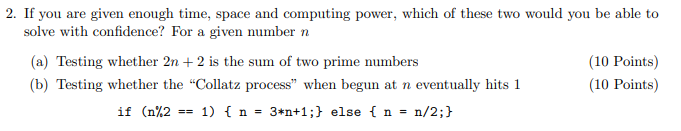Home / Answered Questions / Other / 2-if-you-are-given-enough-time-space-and-computing-power-which-of-these-two-would-you-be-able-to-sol-aw426

# (Solved): 2. If You Are Given Enough Time, Space And Computing Power, Which Of These Two Would You Be Able To ...2. If you are given enough time, space and computing power, which of these two would you be able to solve with confidence? For a given number n (a) Testing whether 2n + 2 is the sum of two prime numbers (10 Points) (b) Testing whether the "Collatz process" when begun at n eventually hits 1 (10 Points) if (n%2 == 1) { n = 3*n+1;} else { n = n/2;}

We have an Answer from Expert